# Some Basic Measures

Regardless of the measurement scale, when the data are gathered we need to analyze them to extract meaningful information. Various measures and statistics are available for summarizing the raw data and for making comparisons across groups. In this section we discuss some basic measures such as ratio, proportion, percentage, and rate, which are frequently used in our daily lives as well as in various activities associated with software development and software quality. These basic measures, while seemingly easy, are often misused. There are also numerous sophisticated statistical techniques and methodologies that can be employed in data analysis. However, such topics are not within the scope of this discussion.

Ratio

A ratio results from dividing one quantity by another. The numerator and denominator are from two distinct populations and are mutually exclusive. For example, in demography, sex ratio is defined as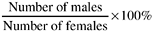If the ratio is less than 100, there are more females than males; otherwise there are more males than females.

Ratios are also used in software metrics. The most often used, perhaps, is the ratio of number of people in an independent test organization to the number of those in the development group. The test/development head-count ratio could range from 1:1 to 1:10 depending on the management approach to the software development process. For the large-ratio (e.g., 1:10) organizations, the development group usually is responsible for the complete development (including extensive development tests) of the product, and the test group conducts system-level testing in terms of customer environment verifications. For the small-ratio organizations, the independent group takes the major responsibility for testing (after debugging and code integration) and quality assurance.

Proportion

Proportion is different from ratio in that the numerator in a proportion is a part of the denominator: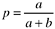Proportion also differs from ratio in that ratio is best used for two groups, whereas proportion is used for multiple categories (or populations) of one group. In other words, the denominator in the preceding formula can be more than just a + b . Ifthen we have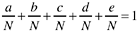When the numerator and the denominator are integers and represent counts of certain events, then p is also referred to as a relative frequency. For example, the following gives the proportion of satisfied customers of the total customer set: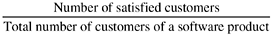The numerator and the denominator in a proportion need not be integers. They can be frequency counts as well as measurement units on a continuous scale (e.g., height in inches, weight in pounds ). When the measurement unit is not integer, proportions are called fractions.

Percentage

A proportion or a fraction becomes a percentage when it is expressed in terms of per hundred units (the denominator is normalized to 100). The word percent means per hundred. A proportion p is therefore equal to 100 p percent (100 p %).

Percentages are frequently used to report results, and as such are frequently misused. First, because percentages represent relative frequencies, it is important that enough contextual information be given, especially the total number of cases, so that the readers can interpret the information correctly. Jones (1992) observes that many reports and presentations in the software industry are careless in using percentages and ratios. He cites the example:

Requirements bugs were 15% of the total, design bugs were 25% of the total, coding bugs were 50% of the total, and other bugs made up 10% of the total.

The project consists of 8 thousand lines of code (KLOC). During its development a total of 200 defects were detected and removed, giving a defect removal rate of 25 defects per KLOC. Of the 200 defects, requirements bugs constituted 15%, design bugs 25%, coding bugs 50%, and other bugs made up 10%.

A second important rule of thumb is that the total number of cases must be sufficiently large enough to use percentages. Percentages computed from a small total are not stable; they also convey an impression that a large number of cases are involved. Some writers recommend that the minimum number of cases for which percentages should be calculated is 50. We recommend that, depending on the number of categories, the minimum number be 30, the smallest sample size required for parametric statistics. If the number of cases is too small, then absolute numbers , instead of percentages, should be used. For instance,

Of the total 20 defects for the entire project of 2 KLOC, there were 3 requirements bugs, 5 design bugs, 10 coding bugs, and 2 others.

When results in percentages appear in table format, usually both the percentages and actual numbers are shown when there is only one variable. When there are more than two groups, such as the example in Table 3.1, it is better just to show the percentages and the total number of cases ( N ) for each group. With percentages and N known, one can always reconstruct the frequency distributions. The total of 100.0% should always be shown so that it is clear how the percentages are computed. In a two-way table, the direction in which the percentages are computed depends on the purpose of the comparison. For instance, the percentages in Table 3.1 are computed vertically (the total of each column is 100.0%), and the purpose is to compare the defect-type profile across projects (e.g., project B proportionally has more requirements defects than project A).

In Table 3.2, the percentages are computed horizontally. The purpose here is to compare the distribution of defects across projects for each type of defect. The inter-pretations of the two tables differ . Therefore, it is important to carefully examine percentage tables to determine exactly how the percentages are calculated.

Table 3.1. Percentage Distributions of Defect Type by Project

Type of Defect

Project A (%)

Project B (%)

Project C (%)

Requirements

115.0

141.0

120.3

Design

125.0

121.8

122.7

Code

150.0

128.6

136.7

Others

110.0

118.6

120.3

Total

100.0

100.0

100.0

( N )

(200)

(105)

(128)

Table 3.2. Percentage Distributions of Defects Across Project by Defect Type

Project

Type of Defect

A

B

C

Total

(N)

Requirements (%)

30.3

43.4

26.3

100.0

(99)

Design (%)

49.0

22.5

28.5

100.0

(102)

Code (%)

56.5

16.9

26.6

100.0

(177)

Others (%)

36.4

16.4

47.2

100.0

(55)

Rate

Ratios, proportions, and percentages are static summary measures. They provide a cross-sectional view of the phenomena of interest at a specific time. The concept of rate is associated with the dynamics (change) of the phenomena of interest; generally it can be defined as a measure of change in one quantity ( y ) per unit of another quantity ( x ) on which the former ( y ) depends. Usually the x variable is time. It is important that the time unit always be specified when describing a rate associated with time. For instance, in demography the crude birth rate (CBR) is defined as:where B is the number of live births in a given calendar year, P is the mid-year population, and K is a constant, usually 1,000.

The concept of exposure to risk is also central to the definition of rate, which distinguishes rate from proportion. Simply stated, all elements or subjects in the denominator have to be at risk of becoming or producing the elements or subjects in the numerator. If we take a second look at the crude birth rate formula, we will note that the denominator is mid-year population and we know that not the entire population is subject to the risk of giving birth. Therefore, the operational definition of CBR is not in compliance with the concept of population at risk, and for this reason, it is a "crude" rate. A better measurement is the general fertility rate, in which the denominator is the number of women of childbearing age, usually defined as ages 15 to 44. In addition, there are other more refined measurements for birth rate.

In literature about quality, the risk exposure concept is defined as opportunities for error (OFE). The numerator is the number of defects of interest. Therefore,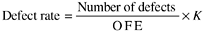In software, defect rate is usually defined as the number of defects per thousand source lines of code (KLOC or KSLOC) in a given time unit (e.g., one year after the general availability of the product in the marketplace , or for the entire life of the product). Note that this metric, defects per KLOC, is also a crude measure. First, the opportunity for error is not known. Second, while any line of source code may be subject to error, a defect may involve many source lines. Therefore, the metric is only a proxy measure of defect rate, even assuming no other problems. Such limitations should be taken into account when analyzing results or interpreting data pertaining to software quality.

Six Sigma

The term six sigma represents a stringent level of quality. It is a specific defect rate: 3.4 defective parts per million (ppm). It was made known in the industry by Motorola, Inc., in the late 1980s when Motorola won the first Malcolm Baldrige National Quality Award (MBNQA). Six sigma has become an industry standard as an ultimate quality goal.

Sigma ( s ) is the Greek symbol for standard deviation. As Figure 3.2 indicates, the areas under the curve of normal distribution defined by standard deviations are constants in terms of percentages, regardless of the distribution parameters. The area under the curve as defined by plus and minus one standard deviation (sigma) from the mean is 68.26%. The area defined by plus/minus two standard deviations is 95.44%, and so forth. The area defined by plus/minus six sigma is 99.9999998%. The area outside the six sigma area is thus 100% -99.9999998% = 0.0000002%.

Figure 3.2. Areas Under the Normal CurveIf we take the area within the six sigma limit as the percentage of defect-free parts and the area outside the limit as the percentage of defective parts, we find that six sigma is equal to 2 defectives per billion parts or 0.002 defective parts per million. The interpretation of defect rate as it relates to the normal distribution will be clearer if we include the specification limits in the discussion, as shown in the top panel of Figure 3.3. Given the specification limits (which were derived from customers' requirements), our purpose is to produce parts or products within the limits. Parts or products outside the specification limits do not conform to requirements. If we can reduce the variations in the production process so that the six sigma (standard deviations) variation of the production process is within the specification limits, then we will have six sigma quality level.

Figure 3.3. Specification Limits, Centered Six Sigma, and Shifted (1.5 Sigma) Six Sigma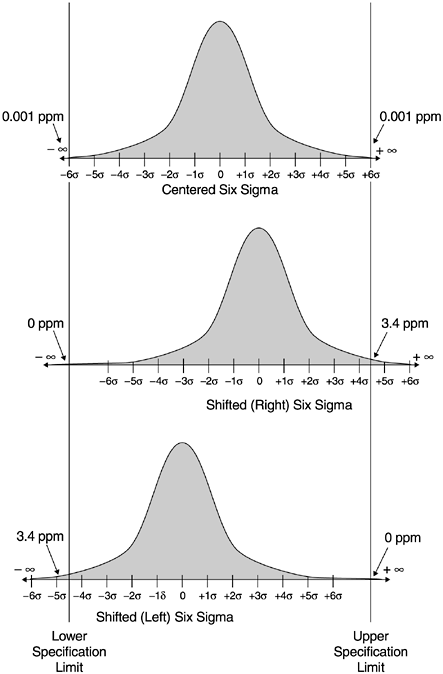The six sigma value of 0.002 ppm is from the statistical normal distribution. It assumes that each execution of the production process will produce the exact distribution of parts or products centered with regard to the specification limits. In reality, however, process shifts and drifts always result from variations in process execution. The maximum process shifts as indicated by research (Harry, 1989) is 1.5 sigma. If we account for this 1.5-sigma shift in the production process, we will get the value of 3.4 ppm. Such shifting is illustrated in the two lower panels of Figure 3.3. Given fixed specification limits, the distribution of the production process may shift to the left or to the right. When the shift is 1.5 sigma, the area outside the specification limit on one end is 3.4 ppm, and on the other it is nearly zero.

The six sigma definition accounting for the 1.5-sigma shift (3.4 ppm) proposed and used by Motorola (Harry, 1989) has become the industry standard in terms of six sigma “level quality (versus the normal distribution's six sigma of 0.002 ppm). Furthermore, when the production distribution shifts 1.5 sigma, the intersection points of the normal curve and the specification limits become 4.5 sigma at one end and 7.5 sigma at the other. Since for all practical purposes, the area outside 7.5 sigma is zero, one may say that the Motorola Six Sigma is equal to the one-tailed 4.5 sigma of the centered normal distribution.

The subtle difference between the centered six sigma and the shifted six sigma may imply something significant. The former is practically equivalent to zero defects, which may invite the debate whether it is feasible to achieve such a goal. The shifted six sigma, while remaining at a very stringent level, does contain a sense of reality. As an example to illustrate the difference, assume we are to clean a house of 1500 sq. ft. By centered six sigma, the area that we allow not to be clean enough is about the area of the head of a pin. By shifted six sigma, the area is about the size of the bottom of a soft drink can. Table 3.3 shows the defect rates by sigma level with and without the 1.5-sigma shift. The defect rates are expressed in terms of defective parts per million (DPPM).

So far our discussion of six sigma has centered on the fact that it is a specific defect rate. Its concept, however, is much richer than that. As we touched on in the discussion, in order to reach six sigma, we have to improve the process. Specifically, we must reduce process variations so that the six sigma variation is still within the specification limits. The notion of process improvement/process variation reduction is, therefore, an inherent part of the concept. Another notion is that of product design and product engineering. If failure tolerance is incorporated into the design of the product, it is a lot easier to make the finished product meet the specifications and, therefore, easier to achieve six sigma quality. The concept of process variation reduction also involves the theory and elaboration of process capability. For details, see Harry and Lawson (1992) and other Motorola literature on the subject (e.g, Smith, 1989). In recent years , the concept and approach of six sigma has been expanded and applied to the improvement of management systems and total quality management. In their recent work, Harry and Schroeder (2000) discuss this expanded approach and its successful applications in several well-known corporations. In Customer-Centered Six Sigma, Naumann and Hoisington (2001) discuss the approach and methods to link six sigma quality and process improvement with customer satisfaction, customer loyalty, and financial results.

Table 3.3. DPPM by Sigma Level with and without Process Shift

Sigma

DPPM (Centered)

DPPM (with 1.5-Sigma Shift)

2

45,500

308,733

3

2,200

66,810

3.5

466

22,700

4

63

6,210

4.5

6.8

1,350

5

0.57

233

5.5

0.038

32

6

0.002

3.4

In software, a defect is a binary variable (the program either works or does not), and it is difficult to relate to continuous distributions such as the normal distribution. However, for discrete distributions there is an equivalent approximation to the six sigma calculation in statistical theory. Moreover, the notions of process improvement and tolerance design cannot be more applicable . In the software industry, six sigma in terms of defect level is defined as 3.4 defects per million lines of code of the software product over its life. Interestingly, the original reason for using the sigma scale to measure quality was to facilitate comparisons across products or organizations. However, in reality this is not the case because the operational definition differs across organizations. For instance, the lines of code in the denominator are taken as the count of shipped source instructions by the International Business Machine Corporation regardless of the language type used to develop the software. Motorola, on the other hand, operationalized the denominator as Assembler language “equivalent instructions. In other words, the normalized lines of code (to Assembler language) is used. To achieve the normalization, the ratios of high-level language to Assembler by Jones (1986) were used. The difference between the two operational definitions can be orders of magnitude. For example, according to Jones's conversion table, one line of PL/I code is equivalent to four lines of Assembler statements, and one line of Smalltalk is equivalent to 15 lines of Assembler.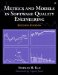Metrics and Models in Software Quality Engineering (2nd Edition)
ISBN: 0201729156
EAN: 2147483647
Year: 2001
Pages: 176